Normal view

# Statistical techniques in business and economics / Robert D. Mason, Douglas A. Lind.

Material type:TextLanguage: English Publication details: c1996. Edition: 9th edDescription: xix, 871 p. : ill. (some col.) ; 26 cmISBN: 0256139016; 0256189048 (instructor's ed.); 9780256139013DDC classification: 519.5 LOC classification: HA29 | .M268 1996Online resources: WorldCat details | E-book Fulltext
Contents:
TOC What is statistics? -- Summarizing data: frequency distributions and graphic presentation -- Describing date--measures of central tendency -- Measures of dispersion and skewness -- A survey of probability concepts -- Discrete probability distributions -- The normal probability distribution -- Sampling methods and sampling distributions -- Tests of hypotheses: large samples -- Tests of hypotheses: small samples -- Analysis of variance -- Linear regression and correlation -- Multiple regression and correlation -- Nonparametric methods: chi-square applications -- Nonparametric methods: analysis of ranked data. Statistical quality control -- Index numbers -- Time series and forecasting -- An introduction to decision making under uncertainty.
Summary: This survey of statistical tools and methods presents concepts in a step-by-step format, illustrating each concept with examples. Features of the text include self-review boxes, integrated coverage Read more...
Tags from this library: No tags from this library for this title.
Star ratingsAverage rating: 0.0 (0 votes)
Holdings
Item type Current library Collection Call number Copy number Status Date due Barcode Item holdsE-Book
E-book
Non-fiction 519.5 MAS 1996 (Browse shelf(Opens below)) Not For LoanText
519.5 MAS (Browse shelf(Opens below)) C-1 Available 1669-1015Text
Reserve Section
Non-fiction 519.5 MAS 1996 (Browse shelf(Opens below)) C-2 Not For Loan 1016Text
Circulation Section
Non-fiction 519.5 MAS 1996 (Browse shelf(Opens below)) C-3 Available 6066
Total holds: 0
##### Browsing EWU Library shelves, Shelving location: E-book Close shelf browser (Hides shelf browser)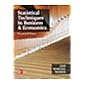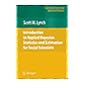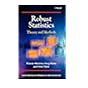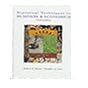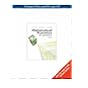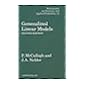519.5 LIS 2018 Statistical techniques in business & economics / 519.5 LYI 2007 Introduction to applied Bayesian statistics and estimation for social scientists / 519.5 MAR 2006 Robust statistics : 519.5 MAS 1996 Statistical techniques in business and economics / 519.5 MAT 2008 Mathematical statistics with applications / 519.5 MCG 1989 Generalized linear models / 519.5 MIJ 2014 John E. Freund's mathematical statistics with applications /

"Key formulas" [4 p.] inserted.

Includes bibliographical references and index.

TOC What is statistics? --
Summarizing data: frequency distributions and graphic presentation --
Describing date--measures of central tendency --
Measures of dispersion and skewness --
A survey of probability concepts --
Discrete probability distributions --
The normal probability distribution --
Sampling methods and sampling distributions --
Tests of hypotheses: large samples --
Tests of hypotheses: small samples --
Analysis of variance --
Linear regression and correlation --
Multiple regression and correlation --
Nonparametric methods: chi-square applications --
Nonparametric methods: analysis of ranked data. Statistical quality control --
Index numbers --
Time series and forecasting --
An introduction to decision making under uncertainty.

This survey of statistical tools and methods presents concepts in a step-by-step format, illustrating each concept with examples. Features of the text include self-review boxes, integrated coverage Read more...

BA

Saifun Momota

There are no comments on this title.